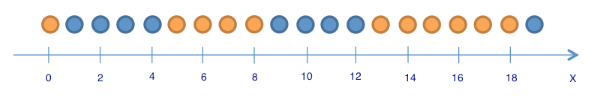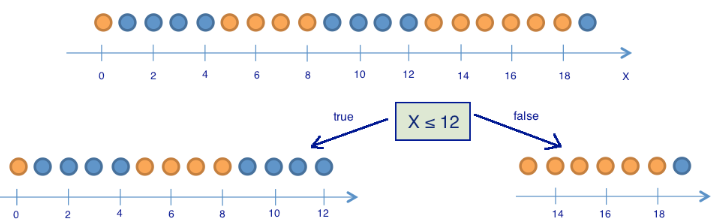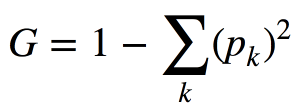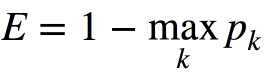### 文章大纲

1. 介绍
2. 决策树
• 如何构建决策树
• 树木构建算法
• 分类问题中裂缝的其他质量标准
• 决策树如何与数字特征一起工作
• 关键树参数
• `DecisionTreeClassifier`在Scikit学习
• 回归问题中的决策树

3.最近邻法

• 真实应用中最近邻方法
• `KNeighborsClassifier`在Scikit学习

4.选择模型参数和交叉验证

5.应用实例和复杂案例

• 客户流失预测任务中的决策树和最近邻法
• 决策树的复杂案例
• MNIST手写数字识别任务中的决策树和k-NN
• 最近邻法的复杂案例

6.决策树的优缺点和最近邻法

7.作业＃3

8.有用的资源

### 1.简介

A computer program is said to learn from experience E with respect to some class of tasks T and performance measure P, if its performance at tasks in T, as measured by P, improves with experience E.。

• 根据其特征将实例分类到其中一个类别;
• 回归 - 基于实例的其他特征预测数值目标特征;
• 聚类 - 根据这些实例的特征识别实例的分组，以便组内的成员彼此更相似，而不是其他组中的成员;
• 异常检测 - 搜索与样本的其余部分或某些实例组“非常不同”的实例;
• 等等。

“深度学习”中的“机器学习基础”一章提供了很好的概述（Ian Goodfellow，Yoshua Bengio，Aaron Courville，2016）。

### 如何构建决策树

#### 熵

Shannon的熵是针对具有N种可能状态的系统定义的，如下所示：

#### 玩具示例来源（俄文）来源（俄文）

### 分类问题中裂缝的其他质量标准基尼系数不确定性（基尼杂质）错误分类错误

#### 例

``````# first class
np.random.seed(17)
train_data = np.random.normal(size=(100, 2))
train_labels = np.zeros(100)
# adding second class
train_data = np.r_[train_data, np.random.normal(size=(100, 2), loc=2)]
train_labels = np.r_[train_labels, np.ones(100)]
view raw
``````

``````plt.rcParams['figure.figsize'] = (10,8)
plt.scatter(train_data[:, 0], train_data[:, 1], c=train_labels, s=100,
cmap='autumn', edgecolors='black', linewidth=1.5);
plt.plot(range(-2,5), range(4,-3,-1));
``````

``````from sklearn.tree import DecisionTreeClassifier

# Let’s write an auxiliary function that will return grid for further visualization.
def get_grid(data):
x_min, x_max = data[:, 0].min() - 1, data[:, 0].max() + 1
y_min, y_max = data[:, 1].min() - 1, data[:, 1].max() + 1
return np.meshgrid(np.arange(x_min, x_max, 0.01), np.arange(y_min, y_max, 0.01))

clf_tree = DecisionTreeClassifier(criterion='entropy', max_depth=3,
random_state=17)

# training the tree
clf_tree.fit(train_data, train_labels)

# some code to depict separating surface
xx, yy = get_grid(train_data)
predicted = clf_tree.predict(np.c_[xx.ravel(),
yy.ravel()]).reshape(xx.shape)
plt.pcolormesh(xx, yy, predicted, cmap='autumn')
plt.scatter(train_data[:, 0], train_data[:, 1], c=train_labels, s=100,
cmap='autumn', edgecolors='black', linewidth=1.5);
``````

``````# use .dot format to visualize a tree
from ipywidgets import Image
from io import StringIO
import pydotplus #pip install pydotplus
from sklearn.tree import export_graphviz
dot_data = StringIO()
export_graphviz(clf_tree, feature_names=['x1', 'x2'],
out_file=dot_data, filled=True)
graph = pydotplus.graph_from_dot_data(dot_data.getvalue())
Image(value=graph.create_png())
view raw
``````

### 决策树如何与数字特征一起工作

``````data = pd.DataFrame({'Age': [17,64,18,20,38,49,55,25,29,31,33],
'Loan Default': [1,0,1,0,1,0,0,1,1,0,1]})
# Let's sort it by age in ascending order.
data.sort_values('Age')
``````
``````age_tree = DecisionTreeClassifier(random_state=17)
age_tree.fit(data['Age'].values.reshape(-1, 1), data['Loan Default'].values)
dot_data = StringIO()
export_graphviz(age_tree, feature_names=['Age'],
out_file=dot_data, filled=True)
graph = pydotplus.graph_from_dot_data(dot_data.getvalue())
Image(value=graph.create_png())
``````

``````data2 = pd.DataFrame({'Age':  [17,64,18,20,38,49,55,25,29,31,33],
'Salary': [25,80,22,36,37,59,74,70,33,102,88],
'Loan Default': [1,0,1,0,1,0,0,1,1,0,1]})
data2.sort_values('Age')
``````

``````age_sal_tree = DecisionTreeClassifier(random_state=17)
age_sal_tree.fit(data2[['Age', 'Salary']].values, data2['Loan Default'].values)
dot_data = StringIO()
export_graphviz(age_sal_tree, feature_names=['Age', 'Salary'],
out_file=dot_data, filled=True)
graph = pydotplus.graph_from_dot_data(dot_data.getvalue())
Image(value=graph.create_png())
``````

### 关键树参数

• 随机森林（一组树）平均构建到最大深度的单个树的响应（稍后我们将讨论为什么要这样做）
• 修剪树木。在这种方法中，树首先被构造成最大深度。然后，从下到上，通过比较具有和不具有该分区的树的质量来移除树的一些节点（使用交叉验证进行比较，更多关于此在下面）。

• 人工限制叶子的深度或最小数量的样本：树的构造在某一点停止;
• 修剪树。

### Scikit-learn中的类DecisionTreeClassifier

`sklearn.tree.DecisionTreeClassifier`该类的主要参数是：

• `max_depth` - 树的最大深度;
• `max_features`- 用于搜索最佳分区的最大功能数量（这对于大量功能来说是必需的，因为搜索所有功能的分区是“昂贵的” ）;
• `min_samples_leaf` - 叶子中的最小样本数量。此参数可防止创建树，其中任何叶只有少数成员。

(未经同意，请勿转载)微信扫一扫关注该公众号